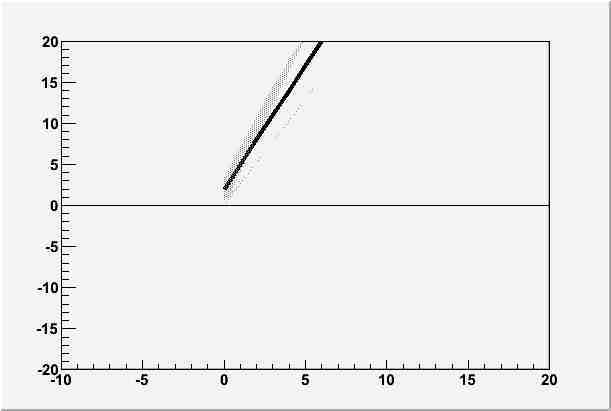# Error Band and User Defined Range

Hi,

I am using TGraphAsymmErrors and I would like to be able to set both my x and y range. Changing the x range seems to work fine. But when I change the y range the error band goes haywire. This is with root version 5.12.00.

Here is a simple example

``````#define BINS 9

Int_t test()
{
TVectorD * x= new TVectorD(BINS);
TVectorD * y= new TVectorD(BINS);
TVectorD * xe= new TVectorD(BINS);
TVectorD  ye;

for(Int_t ie=0; ie < 2; ie++ )
ye[ie]=new TVectorD(BINS);

for(Int_t ix=0; ix < BINS; ix++ )
{
x(ix)=ix;
y(ix)=3*ix+2;
xe(ix)=0;
for(Int_t ie=0; ie < 2; ie++ )
ye[ie][ix]=sqrt(y(ix));

}

TGraphAsymmErrors * gr = new TGraphAsymmErrors(*x,*y,*xe,*xe,ye,ye);
gr->SetFillColor(14);
gr->SetFillStyle(3002);
gr->GetXaxis()->SetLimits(-10,20);
gr->GetHistogram()->SetMaximum(20);
gr->GetHistogram()->SetMinimum(-20);
gr->Draw("3CA");

}
``````

I have attached the resulting graph.test.ps (8.92 KB)

I suggest to simplify your code as shown below

Rene

#define BINS 9

```Int_t bins9() { TCanvas *c1 = new TCanvas("c1"); c1->DrawFrame(-10,-20,20,3*BINS+3); TGraphAsymmErrors * gr = new TGraphAsymmErrors(BINS); for(Int_t ix=0; ix <BINS>SetPoint(ix,ix,y); gr->SetPointError(ix,0,0,sqrt(y),sqrt(y)); } gr->SetFillColor(14); gr->SetFillStyle(3002); gr->Draw("3C"); } ```

Thanks for this great tip DrawFrame is great and I use it all the time.

Alex